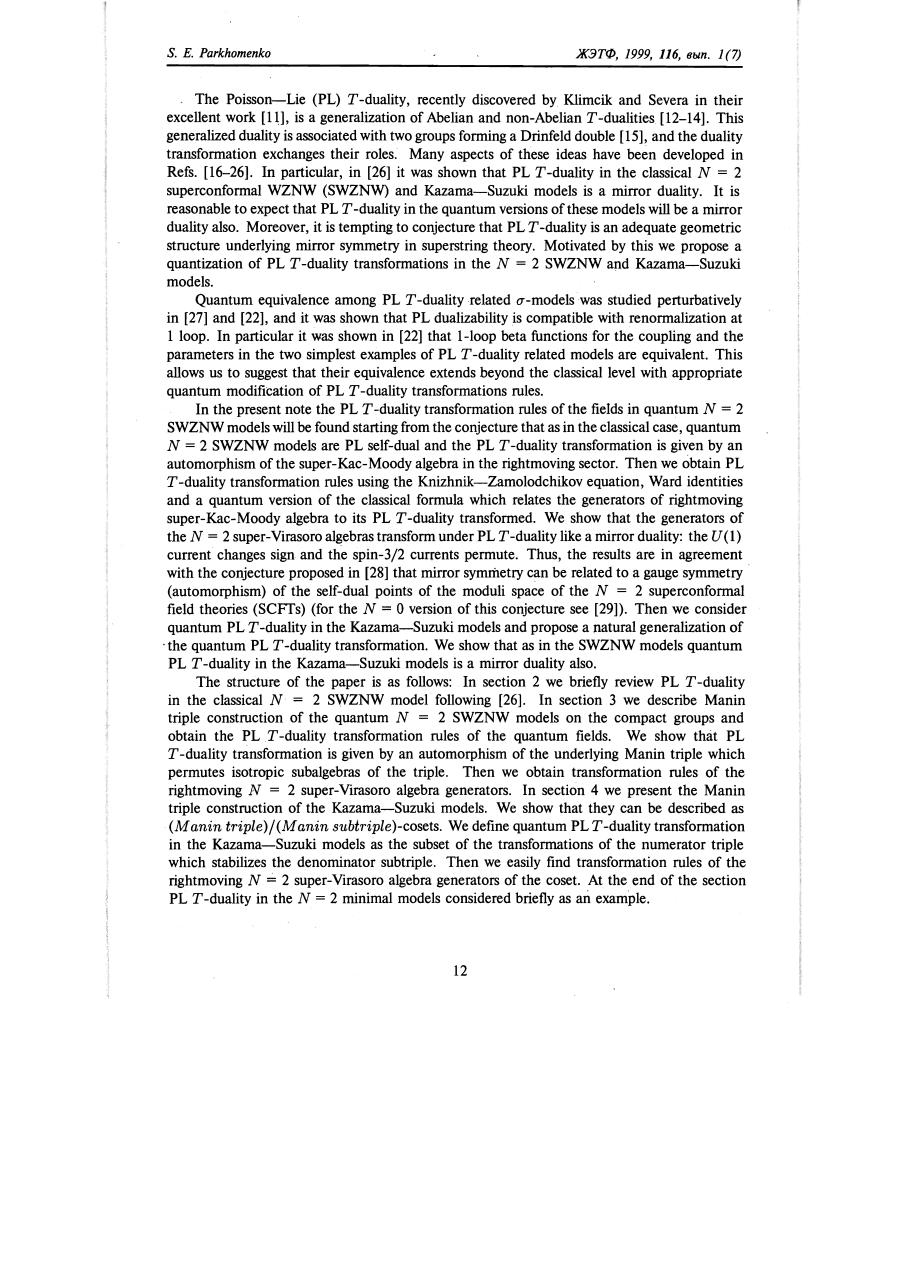# r 116 0011.pdfPage 1 23415

#### Text preview

S.

Е. Parkhoтenko

ЖЭТФ,

1999, 116,

выn.

1 (7)

ТЬе Poisson-Lie (PL) Т -duality, recently discovered Ьу Кlimcik and Severa in their
excellent work , is а generalization of АЬеНаn and non-АЬеНаn T-dualities [12-14]. This
generalized duality is associated with two groups forming а Drinfeld double , and the duality
transformation exchanges their roles. Маnу aspects of these ideas Ьауе Ьееn developed in
Refs. [16-26]. In particular, in  it was shown that PL T-duality in the classical N = 2
superconformal WZNW (SWZNW) and Kazama-Suzuki models is а mirror duality. It is
reasonable to expect that PL Т -duality in the quantum versions of these models will Ье а rnirror
dualityalso. Moreover, it is tempting to conjecture that PL Т -duality is аn adequate geometric
structure underlying rnirror symmetry in superstring theory. Motivated Ьу this we propose а
quantization of PL Т -duality transformations in the N = 2 SWZNW and Kazama-Suzuki
models.
Quantum equivalence among PL Т -duality related O'-modelswas studied perturbatively
in  and , and it was shown that PL dualizability is compatible with renormalization at
1 loop. In particular it was shown in  that 1-100p beta functions for the coupling and the
parameters in the two simplest examples of PL Т -duality related mode1s are equivalent. This
allows us to suggest that their equivalence extends beyond the classical level with appropriate
quantum modifkation of PL Т -duality transformations rules.
In the present note the PL Т -duality transformation rules of the fields in quantum N = 2
SWZNW mode1s will Ье found starting from the conjecture that as in the classical case, quantum
N = 2 SWZNW models are PL self-dual and the PL Т -duality transformation is given Ьу аn
automorphism ofthe super-Kac-Moody algebra in the rightmoving sector. ТЬеn we obtain PL
т -duality transformation rules using the Кnizhnik-Zamolodchikov equation, Ward identities
and а quantum version of the classical formula which relates the generators of rightmoving
super- Кас- Moody algebra to its PL Т -duality transformed. We show that the generators of
the N = 2 super-Virasoro algebras transform under PL Т -duality like а rnirror duality: the и (1)
current changes sign and the sрin-З/2 currents permute. Thus, the results are in agreement
with the conjecture proposed in  that rnirror symriletry сап Ье related to а gauge symmetry
(automorphism) of the self-dual points of the moduli space of the N = 2 superconformal
field theories (SCFТs) (for the N = О version of this conjecture see ). ТЬеn we consider
quantum PL Т -duality in the Kazama-Suzuki models and propose а natural generalization of
. the quantum PL Т -duality transformation. We show that as in the SWZNW models quantum
PL т -duality in the Kazama-Suzuki models is а mirror duality also.
ТЬе structure of the paper is as follows: In section 2 we briefly review PL Т -duality
in the classical N = 2 SWZNW model following . In section 3 we describe Manin
triple construction of the quantum N = 2 SWZNW models оп the compact groups and
obtain the PL Т -duality transformation rules of the quantum fields. We show that PL
т -duality transformation is given Ьу аn automorphism of the underlying Manin triple which
permutes isotropic subalgebras of the triple. ТЬеn we obtain transformation rules of the
rightmoving N = 2 super-Virasoro algebra generators. In section 4 we present (Ье Manin
triple construction of the Кazama-Suzuki models. We show that they сап Ье described as
(М anin triple)j(M anin subtriple)-cosets. We define quantum PL T-duality transformation
in the Kazama-Suzuki models as the subset of the transformations of the numerator triple
which stabilizes the denominator subtriple. ТЬеn we easily find transformation rules of the
rightmoving N = 2 super-Virasoro algebra generators of the coset. At the end of the section
PL т -duality in the N = 2 rninimal models considered briefly as ал example.

12